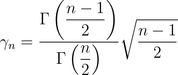# INF7100, statistiques

La seconde partie de mon intervention sur la science des données, dans le cadre du cours INF7100 portera sur les statistiques, univariées et multivarirées. Le plan sera le suivant

• 201: De la Statistique aux Sciences de Données pdf video (14:24)
• 211: Fonctions Usuelles en Statistique (fonction de réparition, densité, histogramme) pdf video (28:37)
• 221: Indicateurs Statistique: Valeur Centrale (moyenne) pdf video (32:56)
• 222: Indicateurs Statistique: Dispersion (variance, inégalités) pdf video (22:21)
• 223: Indicateurs Statistique: Approximations (approximation normale) pdf video (18:42)
• 224: Indicateurs Statistique: Quantiles pdf video (24:54)
• 231: Inférence (statistique bayésienne) pdf video (39:33)
• 241: Tests Statistiques (1) (tests, significativité, p-value) pdf video (43:41)
• 242: Tests Statistiques (2) (erreurs) pdf video (16:51)
• 261: Statistiques Bivariées pdf video (25:16)
• 271: Statistiques Multivariées: Projections pdf video (29:06)
• 272: Statistiques Multivariées: Clusters pdf video (32:21)
• 281: Réseaux et Graphs pdf video (32:40)
• 291: Données Chronologiques pdf video (29:01)

Usually, when I give a course on GLMs, I try to insist on the fact that the link function is probably more important than the distribution. In order to illustrate, consider the following dataset, with 5 observations

x = c(1,2,3,4,5) y = c(1,2,4,2,6) base = data.frame(x,y)

Then consider several model, with various distributions, and either an identity link (and in that case $\mathbb{E}[Y|\mathbf{X}=\mathbf{x}]=\mathbf{x}^T\mathbf{\beta}$) or a log link function (so that $\mathbb{E}[Y|\mathbf{X}=\mathbf{x}]=e^{\mathbf{x}^T\mathbf{\beta}}$)

regNId = glm(y~x,family=gaussian(link="identity"),data=base) regNlog = glm(y~x,family=gaussian(link="log"),data=base) regPId = glm(y~x,family=poisson(link="identity"),data=base) regPlog = glm(y~x,family=poisson(link="log"),data=base) regGId = glm(y~x,family=Gamma(link="identity"),data=base) regGlog = glm(y~x,family=Gamma(link="log"),data=base) regIGId = glm(y~x,family=inverse.gaussian(link="identity"),data=base) regIGlog = glm(y~x,family=inverse.gaussian(link="log"),data=base

One can also consider some Tweedie distribution, to be even more general

library(statmod) regTwId = glm(y~x,family=tweedie(var.power=1.5,link.power=1),data=base) regTwlog = glm(y~x,family=tweedie(var.power=1.5,link.power=0),data=base)

Consider the prediction obtained in the first case, with the linear link function

library(RColorBrewer) darkcols = brewer.pal(8, "Dark2") plot(x,y,pch=19) abline(regNId,col=darkcols) abline(regPId,col=darkcols) abline(regGId,col=darkcols) abline(regIGId,col=darkcols) abline(regTwId,lty=2)The predictions are very very close, aren’t they ? In the case of the exponential prediction, we obtain

plot(x,y,pch=19) u=seq(.8,5.2,by=.01) lines(u,predict(regNlog,newdata=data.frame(x=u),type="response"),col=darkcols) lines(u,predict(regPlog,newdata=data.frame(x=u),type="response"),col=darkcols) lines(u,predict(regGlog,newdata=data.frame(x=u),type="response"),col=darkcols) lines(u,predict(regIGlog,newdata=data.frame(x=u),type="response"),col=darkcols) lines(u,predict(regTwlog,newdata=data.frame(x=u),type="response"),lty=2)We can actually look closer. For instance, in the linear case, consider the slope obtained with a Tweedie model (that will include all the parametric familes mentioned here, actually)

pente=function(gamma) summary(glm(y~x,family=tweedie(var.power=gamma,link.power=1),data=base))$coefficients[2,1:2] Vgamma = seq(-.5,3.5,by=.05) Vpente = Vectorize(pente)(Vgamma) plot(Vgamma,Vpente[1,],type="l",lwd=3,ylim=c(.965,1.03),xlab="power",ylab="slope")The slope here is always very very close to one ! Even more if we add a confidence interval plot(Vgamma,Vpente[1,]) lines(Vgamma,Vpente[1,]+1.96*Vpente[2,],lty=2) lines(Vgamma,Vpente[1,]-1.96*Vpente[2,],lty=2)Heuristically, for the Gamma regression, or the Inverse Gaussian one, because the variance is a power of the prediction, if the prediction is small (here on the left), the variance should be small. So, on the left of the graph, the error should be small with a higher power for the variance function. And that’s indeed what we observe here erreur=function(gamma) predict(glm(y~x,family=tweedie(var.power=gamma,link.power=1),data=base),newdata=data.frame(x=1),type="response")-y[x==1] Verreur = Vectorize(erreur)(Vgamma) plot(Vgamma,Verreur,type="l",lwd=3,ylim=c(-.1,.04),xlab="power",ylab="error") abline(h=0,lty=2)Of course, we can do the same with the exponential models pente=function(gamma) summary(glm(y~x,family=tweedie(var.power=gamma,link.power=0),data=base))$coefficients[2,1:2] Vpente = Vectorize(pente)(Vgamma) plot(Vgamma,Vpente[1,],type="l",lwd=3)or, if we add the confidence bands, we obtain

plot(Vgamma,Vpente[1,],ylim=c(0,.8),type="l",lwd=3,xlab="power",ylab="slope") lines(Vgamma,Vpente[1,]+1.96*Vpente[2,],lty=2) lines(Vgamma,Vpente[1,]-1.96*Vpente[2,],lty=2)So here also, the “slope” is rather similar… And if we look at the error we make on the left part of the graph, we obtain

erreur=function(gamma) predict(glm(y~x,family=tweedie(var.power=gamma,link.power=0),data=base),newdata=data.frame(x=1),type="response")-y[x==1] Verreur = Vectorize(erreur)(Vgamma) plot(Vgamma,Verreur,type="l",lwd=3,ylim=c(.001,.32),xlab="power",ylab="error")So my point is that the distribution is usually not the most important point on GLMs, even if chapters of books on GLMs are distribution based… But as mentioned in an another post, if you consider a nonlinear transformation, like we have with GAMs, the story is more complicated…

# Unbiased Estimators vs. Minimizing a Quadratic Loss Function

Unbiased estimators are important in statistics. I guess because of Cramér Rao bound, for the variance. In the sense that if  , then  , where   denotes Fisher information (the proof was writen in an old post).

But what could we be the variance if   is not unbiased ?

Consider the following simple case, with a Gaussian i.id. sample   from a  . We know that the estimator of the Method of Moments is the same as the Maximum Likelihood estimator, i.e.  . And this estimator is efficient, in the sense that its variance is equal to Cramér-Rao lower bound,  .

But what if we consider another estimator? For instance  , with   not necessarily equal to 1. In that case, this estimator is (usually) biased since

And its variance is

We can visualise those two functions (the bias and the variance) using

 n=10 alpha=seq(0,2,by=.01) b=1-alpha v=alpha^2/n plot(alpha,b,xlab="alpha",col="red",type="l") par(new=TRUE) plot(alpha,v,col="blue",type="l",axes=FALSE axis(4,) mtext("bias",side=2,line=-1,col="red") mtext("variance",side=4,line=-1,col="blue")Observe that if is small (smaller than 1), the variance is smaller than the Cramér-Rao lower bound. And here, the mean squared error, defined as

which is, here,

The optimal value is obtained when the first order condition is satisfied

i.e.

So biased estimators can be more interesting than unbiased estimators, if the goal is the minimize the mean square error.

# Heuristics on bias and variance for kernel density estimators

Consider the simple case of a moving histogram (which is a very simple kernel). The idea is to recall that

where

is the slope close to point .Then we use the empirical cumulative density to approximate the slope, i.e.

which can also be writen

Consider now the density seen as a random variable

where the‘s are i.i.d. where , with

Thus, observe that , but that’s not what we’re looking for… From Taylor’s expansion,

thus

where the bias comes from the approximation of the density by some string. About the variance,

thus, since ,

i.e.

We can observe that

is decreasing as , while the variance is increasing as . This is the standard bias-variance tradeoff in statistics.

# Variance of the Average of a Sequence

In the case where  are i.i.d. random variables, then

Now, what if  are identically distributed, but no longer independent. What if we have an autoregressive process? Assume that

Then

can be written

Here, we will express the variance as a function of  and , but it is possible to use also , since, in the context of an ,

Now, since  we get

which can be simplified, since

i.e.

So, the variance of the mean can be writen as

Observe that if  is large enough,

This asymptotic relationship is well known actually. A simple way to get it is the following. One can can write

or equivalently

But actually, the first relationship is probably more interesting to get an asymptotic approximation,

In the context of an  process, this can be writen

Thus, we get the following well-known relationship

In the case where  is an i.i.d. sequence, i.e. , then we get the relationship mentioned initially. And in the case of a random walk… unfortunately, we cannot use that relationship. But observe that

i.e.

which can be written

If we compare the true value and the approximation, we get the following graph,

> V=function(phi,s2=1,n=100){
+ g0=s2/(1-phi^2)
+ if(phi<1){
+ if(phi==0){v1=g0/n}
+ if(phi>0){v1=g0/n^2*(n+2*((n-1)*
+ phi^(-1)-n+phi^(n-1))/(phi^(-1)-1)^2)}
+ v2=g0/n*(1+phi)/(1-phi)
+ }
+ if(phi==1){
+ v1=(2*n+1)*(n+1)*s2/(6*n)
+ v2=NA
+ }
+ return(c(v1,v2))}
>
> Vphi=function(phi) V(phi,1,100)
> x=seq(.01,1,by=.02)
> M=matrix(unlist(lapply(x,V)),nrow=2)
> plot(x,M[1,],type="l",col="red",log="y",
+ ylab="Variance of the average (log scale)",
+ xlab="Autoregressive coefficient")
> lines(x,M[2,],col="blue")# Visualizing overdispersion (with trees)

This week, we started to discuss overdispersion when modeling claims frequency. In my previous post, I discussed computations of empirical variances with different exposure. But I did use only one factor to compute classes. Of course, it is possible to use much more factors. For instance, using cartesian products of factors,

> X=as.factor(paste(sinistres$carburant,sinistres$zone,
+ cut(sinistres$ageconducteur,breaks=c(17,24,40,65,101)))) > E=sinistres$exposition
> Y=sinistres$nbre > vm=vv=ve=rep(NA,length(levels(X))) > for(i in 1:length(levels(X))){ + ve[i]=Ei=E[X==levels(X)[i]] + Yi=Y[X==levels(X)[i]] + vm[i]=meani=weighted.mean(Yi/Ei,Ei) # moyenne + vv[i]=variancei=sum((Yi-meani*Ei)^2)/sum(Ei) # variance + cat("Class ",levels(X)[i],"average =",meani," variance =",variancei,"\n") + } Class D A (17,24] average = 0.06274415 variance = 0.06174966 Class D A (24,40] average = 0.07271905 variance = 0.07675049 Class D A (40,65] average = 0.05432262 variance = 0.06556844 Class D A (65,101] average = 0.03026999 variance = 0.02960885 Class D B (17,24] average = 0.2383109 variance = 0.2442396 Class D B (24,40] average = 0.06662015 variance = 0.07121064 Class D B (40,65] average = 0.05551854 variance = 0.05543831 Class D B (65,101] average = 0.0556386 variance = 0.0540786 Class D C (17,24] average = 0.1524552 variance = 0.1592623 Class D C (24,40] average = 0.0795852 variance = 0.09091435 Class D C (40,65] average = 0.07554481 variance = 0.08263404 Class D C (65,101] average = 0.06936605 variance = 0.06684982 Class D D (17,24] average = 0.1584052 variance = 0.1552583 Class D D (24,40] average = 0.1079038 variance = 0.121747 Class D D (40,65] average = 0.06989518 variance = 0.07780811 Class D D (65,101] average = 0.0470501 variance = 0.04575461 Class D E (17,24] average = 0.2007164 variance = 0.2647663 Class D E (24,40] average = 0.1121569 variance = 0.1172205 Class D E (40,65] average = 0.106563 variance = 0.1068348 Class D E (65,101] average = 0.1572701 variance = 0.2126338 Class D F (17,24] average = 0.2314815 variance = 0.1616788 Class D F (24,40] average = 0.1690485 variance = 0.1443094 Class D F (40,65] average = 0.08496827 variance = 0.07914423 Class D F (65,101] average = 0.1547769 variance = 0.1442915 Class E A (17,24] average = 0.1275345 variance = 0.1171678 Class E A (24,40] average = 0.04523504 variance = 0.04741449 Class E A (40,65] average = 0.05402834 variance = 0.05427582 Class E A (65,101] average = 0.04176129 variance = 0.04539265 Class E B (17,24] average = 0.1114712 variance = 0.1059153 Class E B (24,40] average = 0.04211314 variance = 0.04068724 Class E B (40,65] average = 0.04987117 variance = 0.05096601 Class E B (65,101] average = 0.03123003 variance = 0.03041192 Class E C (17,24] average = 0.1256302 variance = 0.1310862 Class E C (24,40] average = 0.05118006 variance = 0.05122782 Class E C (40,65] average = 0.05394576 variance = 0.05594004 Class E C (65,101] average = 0.04570239 variance = 0.04422991 Class E D (17,24] average = 0.1777142 variance = 0.1917696 Class E D (24,40] average = 0.06293331 variance = 0.06738658 Class E D (40,65] average = 0.08532688 variance = 0.2378571 Class E D (65,101] average = 0.05442916 variance = 0.05724951 Class E E (17,24] average = 0.1826558 variance = 0.2085505 Class E E (24,40] average = 0.07804062 variance = 0.09637156 Class E E (40,65] average = 0.08191469 variance = 0.08791804 Class E E (65,101] average = 0.1017367 variance = 0.1141004 Class E F (17,24] average = 0 variance = 0 Class E F (24,40] average = 0.07731177 variance = 0.07415932 Class E F (40,65] average = 0.1081142 variance = 0.1074324 Class E F (65,101] average = 0.09071118 variance = 0.1170159 Again, one can plot the variance against the average, > plot(vm,vv,cex=sqrt(ve),col="grey",pch=19, + xlab="Empirical average",ylab="Empirical variance") > points(vm,vv,cex=sqrt(ve)) > abline(a=0,b=1,lty=2)An alternative is to use a tree. The tree can be obtained from another variable (the insured had, or had not, a claim, during the period considered) but it should be rather close to the one we would like to model (the number of claims over the period considered). Here, I did use the whole database (with more that 600,000 lines) > library(tree) > T=tree((nombre>0)~as.factor(zone)+as.factor(puissance)+ + as.factor(marque)+as.factor(carburant)+as.factor(region)+ + agevehicule+ageconducteur,data=baseFREQ, + split = "gini",minsize =25000) The tree is the following > plot(T) > text(T)Now, each knot defines a class, and it is possible to use it to define a class. Which is supposed to be homogeneous. > X=as.factor(T$where)
> E=sinistres$exposition > Y=sinistres$nbre
> vm=vv=ve=rep(NA,length(levels(X)))
>   for(i in 1:length(levels(X))){
+  	   ve[i]=Ei=E[X==levels(X)[i]]
+  	   Yi=Y[X==levels(X)[i]]
+   vm[i]=meani=weighted.mean(Yi/Ei,Ei)    # moyenne
+   vv[i]=variancei=sum((Yi-meani*Ei)^2)/sum(Ei)    # variance
+  cat("Class ",levels(X)[i],"average =",meani," variance =",variancei,"\n")
+  }
Class  6 average =   0.04010406  variance = 0.04424163
Class  8 average =   0.05191127  variance = 0.05948133
Class  9 average =   0.07442635  variance = 0.08694552
Class  10 average =  0.4143646   variance = 0.4494002
Class  11 average =  0.1917445   variance = 0.1744355
Class  15 average =  0.04754595  variance = 0.05389675
Class  20 average =  0.08129577  variance = 0.0906322
Class  22 average =  0.05813419  variance = 0.07089811
Class  23 average =  0.06123807  variance = 0.07010473
Class  24 average =  0.06707301  variance = 0.07270995
Class  25 average =  0.3164557   variance = 0.2026906
Class  26 average =  0.08705041  variance = 0.108456
Class  27 average =  0.06705214  variance = 0.07174673
Class  30 average =  0.05292652  variance = 0.06127301
Class  31 average =  0.07195285  variance = 0.08620593
Class  32 average =  0.08133722  variance = 0.08960552
Class  34 average =  0.1831559   variance = 0.2010849
Class  39 average =  0.06173885  variance = 0.06573939
Class  41 average =  0.07089419  variance = 0.07102932
Class  44 average =  0.09426152  variance = 0.1032255
Class  47 average =  0.03641669  variance = 0.03869702
Class  49 average =  0.0506601   variance = 0.05089276
Class  50 average =  0.06373107  variance = 0.06536792
Class  51 average =  0.06762947  variance = 0.06926191
Class  56 average =  0.06771764  variance = 0.07122379
Class  57 average =  0.04949142  variance = 0.05086885
Class  58 average =  0.2459016   variance = 0.2451116
Class  59 average =  0.05996851  variance = 0.0615773
Class  61 average =  0.07458053  variance = 0.0818608
Class  63 average =  0.06203737  variance = 0.06249892
Class  64 average =  0.07321618  variance = 0.07603106
Class  66 average =  0.07332127  variance = 0.07262425
Class  68 average =  0.07478147  variance = 0.07884597
Class  70 average =  0.06566728  variance = 0.06749411
Class  71 average =  0.09159605  variance = 0.09434413
Class  75 average =  0.03228927  variance = 0.03403198
Class  76 average =  0.04630848  variance = 0.04861813
Class  78 average =  0.05342351  variance = 0.05626653
Class  79 average =  0.05778622  variance = 0.05987139
Class  80 average =  0.0374993   variance = 0.0385351
Class  83 average =  0.06721729  variance = 0.07295168
Class  86 average =  0.09888492  variance = 0.1131409
Class  87 average =  0.1019186   variance = 0.2051122
Class  88 average =  0.05281703  variance = 0.0635244
Class  91 average =  0.08332136  variance = 0.09067632
Class  96 average =  0.07682093  variance = 0.08144446
Class  97 average =  0.0792268   variance = 0.08092019
Class  99 average =  0.1019089   variance = 0.1072126
Class  100 average = 0.1018262   variance = 0.1081117
Class  101 average = 0.1106647   variance = 0.1151819
Class  103 average = 0.08147644  variance = 0.08411685
Class  104 average = 0.06456508  variance = 0.06801061
Class  107 average = 0.1197225   variance = 0.1250056
Class  108 average = 0.0924619   variance = 0.09845582
Class  109 average = 0.1198932   variance = 0.1209162

Here, when ploting the empirical variance (per knot) against the empirial average of claims, we getHere, we can identify classes where remaining heterogeneity.

# Overdispersion with different exposures

In actuarial science, and insurance ratemaking, taking into account the exposure can be a nightmare (in datasets, some clients have been here for a few years – we call that exposure – while others have been here for a few months, or weeks). Somehow, simple results because more complicated to compute just because we have to take into account the fact that exposure is an heterogeneous variable.The exposure in insurance ratemaking can be seen as a problem of censored data (in my dataset, the exposure is always smaller than 1 since observations are contracts, not policyholders),

• the number of claims  on the period  is unobserved
• the number of claims  on  is observed (as well as )

And as always, the variable of interest is the unobserved one, because we have to price insurance contract with a cover period of one (full) year. So we have to model the yearly frequency of insurance claims.In our dataset, we have ‘s – or more generally also some additional covariates ‘s. For ratemaking, we need to estimate and perhaps also (for instance to test if the Poisson assumption is valid, or not). To estimate the expected value, a natural estimate for  (forget about covariates as a start) is

which is also the weight average of annualized individual counts

We consider the ratio of the total number of claims to the total exposure-to-
risk. This estimate appears for instance if we consider a Poisson process, so that while . Then, the likelihood is

i.e.

The first order condition is here

which is satisfied if

So, we do have an estimator for the expected value, and a natural estimator for  is then (if we consider categorical covariates)

Now, we need an estimate for the variance, or more precisely the conditional variable. Assume (as a starting point) that all have the same exposure . For instance, if is one half, insured were observed only the first six months. Then  with ( is the number of claims on the first six months, while are the number of claims on the last six months), i.e. if we assume independent increments. I.e.
, or conversely . More generally, it is reasonable to assume that

for all values of . And then

Thus, it seems legitimate to assume that the empirical variance of  can be written

Since the average of  is , then

or equivalently
i.e.

Thus, with different ‘s, it would be legitimate (I guess) to consider

Thus, an estimator for is

This can be used to test is the Poisson assumption is valid to model frequency. Consider the following dataset,

>  sinistre=read.table("http://freakonometrics.free.fr/sinistreACT2040.txt",
>  sinistres=sinistre[sinistre$garantie=="1RC",] > sinistres=sinistres[sinistres$cout>0,]
>  T=table(sinistres$nocontrat) > T1=as.numeric(names(T)) > T2=as.numeric(T) > nombre1 = data.frame(nocontrat=T1,nbre=T2) > I = contrat$nocontrat%in%T1
>  T1= contrat$nocontrat[I==FALSE] > nombre2 = data.frame(nocontrat=T1,nbre=0) > nombre=rbind(nombre1,nombre2) > baseFREQ = merge(contrat,nombre) Here, we do have our two variables of interest, the exposure, per contract, > E <- baseFREQ$exposition

and the (observed) number of claims (during that time frame)

>  Y <- baseFREQ$nbre It is possible to compute without covariates, the average (yearly) number of claims, per contract, and the associated variance > (mean=weighted.mean(Y/E,E))  0.07279295 > (variance=sum((Y-mean*E)^2)/sum(E))  0.08778567 It looks like the variance is (slightly) larger than the average (we’ll see in a few weeks how to test it, more formally). It is possible to add covariates, for instance the density of population, in the area where the policyholder lives, > X=as.factor(baseFREQ$densite)
>  for(i in 1:length(levels(X))){
+ 	   Ei=E[X==levels(X)[i]]
+ 	   Yi=Y[X==levels(X)[i]]
+  (meani=weighted.mean(Yi/Ei,Ei))    # moyenne
+  (variancei=sum((Yi-meani*Ei)^2)/sum(Ei))    # variance
+ cat("Density, zone",levels(X)[i],"average =",meani," variance =",variancei,"\n")
+ }
Density, zone 11 average = 0.07962411  variance = 0.08711477
Density, zone 21 average = 0.05294927  variance = 0.07378567
Density, zone 22 average = 0.09330982  variance = 0.09582698
Density, zone 23 average = 0.06918033  variance = 0.07641805
Density, zone 24 average = 0.06004009  variance = 0.06293811
Density, zone 25 average = 0.06577788  variance = 0.06726093
Density, zone 26 average = 0.0688496   variance = 0.07126078
Density, zone 31 average = 0.07725273  variance = 0.09067
Density, zone 41 average = 0.03649222  variance = 0.03914317
Density, zone 42 average = 0.08333333  variance = 0.1004027
Density, zone 43 average = 0.07304602  variance = 0.07209618
Density, zone 52 average = 0.06893741  variance = 0.07178091
Density, zone 53 average = 0.07725661  variance = 0.07811935
Density, zone 54 average = 0.07816105  variance = 0.08947993
Density, zone 72 average = 0.08579731  variance = 0.09693305
Density, zone 73 average = 0.04943033  variance = 0.04835521
Density, zone 74 average = 0.1188611   variance = 0.1221675
Density, zone 82 average = 0.09345635  variance = 0.09917425
Density, zone 83 average = 0.04299708  variance = 0.05259835
Density, zone 91 average = 0.07468126  variance = 0.3045718
Density, zone 93 average = 0.08197912  variance = 0.09350102
Density, zone 94 average = 0.03140971  variance = 0.04672329

Perhaps graphs would be a nice tool to play with, to visualize that information

> plot(meani,variancei,cex=sqrt(Ei),col="grey",pch=19,
+ xlab="Empirical average",ylab="Empirical variance")
> points(meani,variancei,cex=sqrt(Ei))The size of the circles is related to the size of the group (the area is proportional to the total exposure within the group). The first diagonal corresponds to the Poisson model, i.e. the variance should be equal to the mean. It is also possible to consider other covariates, like the gas typeor the car brand,It is also possible to consider the age of the driver as a categorical variateActually, the age is interesting: we can observe on that dataset a feature that Jean-Philippe Boucher observed also on his own datasets. Let us look more carefully where are the different ages,On the right, we can observe young (unexperienced) drivers. That was expected. But some classes are below the first diagonal: the expected frequency is large, but not the variance. I.e. we know for sure that young drivers have more car accidents. It is not an heterogeneous class, on the contrary: young drivers can be seen as a relatively homogeneous class, with a high frequency of car accidents.

With the original dataset (here, I use only a subset with 50,000 clients), we do obtain the following graph:If we do not observe underdispersion for young drivers, observe that those are incredibly homogeneous classes. With a clear impact of experience, since circles are moving downward from age 18 to 25.

Another disturbing story (this was – one more time – suggestion from Jean-Philippe) that it might be possible to consider the exposure as a standard variable, and see if the coefficient is actually equal to 1. Without any covariate,

>  reg=glm(Y~log(E),family=poisson("log"))
>  summary(reg)

Call:
glm(formula = Y ~ log(E), family = poisson("log"))

Deviance Residuals:
Min       1Q   Median       3Q      Max
-0.3988  -0.3388  -0.2786  -0.1981  12.9036

Coefficients:
Estimate Std. Error z value Pr(>|z|)
(Intercept) -2.83045    0.02822 -100.31   <2e-16 ***
log(E)       0.53950    0.02905   18.57   <2e-16 ***
---
Signif. codes:  0 ‘***’ 0.001 ‘**’ 0.01 ‘*’ 0.05 ‘.’ 0.1 ‘ ’ 1

(Dispersion parameter for poisson family taken to be 1)

Null deviance: 12931  on 49999  degrees of freedom
Residual deviance: 12475  on 49998  degrees of freedom
AIC: 16150

Number of Fisher Scoring iterations: 6

i.e. the parameter is clearly strictly smaller than 1. And it is neither related to significance,

> library(car)
> linearHypothesis(reg,"log(E)",1)
Linear hypothesis test

Hypothesis:
log(E) = 1

Model 1: restricted model
Model 2: Y ~ log(E)

Res.Df Df  Chisq Pr(>Chisq)
1  49999
2  49998  1 251.19  < 2.2e-16 ***
---
Signif. codes:  0 ‘***’ 0.001 ‘**’ 0.01 ‘*’ 0.05 ‘.’ 0.1 ‘ ’ 1

nor to the fact that I did not take into account covariates,

> reg=glm(nbre~log(exposition)+carburant+as.factor(ageconducteur)+as.factor(densite),family=poisson("log"),data=baseFREQ)
>  summary(reg)

Call:
glm(formula = nbre ~ log(exposition) + carburant + as.factor(ageconducteur) +
as.factor(densite), family = poisson("log"), data = baseFREQ)

Deviance Residuals:
Min       1Q   Median       3Q      Max
-0.7114  -0.3200  -0.2637  -0.1896  12.7104

Coefficients:
Estimate Std. Error z value Pr(>|z|)
(Intercept)                  -14.07321  181.04892  -0.078 0.938042
log(exposition)                0.56781    0.03029  18.744  < 2e-16 ***
carburantE                    -0.17979    0.04630  -3.883 0.000103 ***
as.factor(ageconducteur)19    12.18354  181.04915   0.067 0.946348
as.factor(ageconducteur)20    12.48752  181.04902   0.069 0.945011

(etc). So it might be a too strong assumption to assume that the exposure is an exogenous variate here. But that’s another story !

# De la difficulté de faire parler les chiffres…

Parution d’un court article intituléde la difficulté de faire parler des chiffres pour analyser la gravité des accidents de la route” dans le dernier numéro de Variance. Le numéro complet est en ligne sur http://ensae.org/…. Sinon, tous les articles de vulgarisation sont en ligne sur http://freakonometrics.hypotheses.org/….

Le code pour le premier graphique (sur les tuées) est

base=read.table(
"http://freakonometrics.free.fr/base-graph-accidents-graves.txt",
base$date=as.Date(base$date)
base$dateavant=as.Date(base$dateavant)
base$dateapres=as.Date(base$dateapres)
plot(base$date,base$compte,main="Blessés graves sur route entre 2002 et 2009",
xlab="Date",
ylab="Nombre de blessés sur la route, par jour",col="white")
points(base$dateavant,base$compteavant,col="light green")
lines(base$dateavant,base$tendanceavant,col="red",lty=2)
lines(base$dateavant,base$splinesavant,lwd=3,col="red")
points(base$dateapres,base$compteapres,col="light blue")
lines(base$dateapres,base$tendanceapres,col="red",lty=2)
lines(base$dateapres,base$splinesapres,lwd=3,col="red")

alors que pour les seconds (sur les blessés)

base=read.table(
"http://freakonometrics.free.fr/base-graph-accidents-deces.txt",
base$date=as.Date(base$date)
base$dateavant=as.Date(base$dateavant)
base$dateapres=as.Date(base$dateapres)
plot(base$date,base$compte,main="Blessés graves sur route entre 2002 et 2009",
xlab="Date",
ylab="Nombre de blessés sur la route, par jour",col="white")
points(base$dateavant,base$compteavant,col="light green")
lines(base$dateavant,base$tendanceavant,col="red",lty=2)
lines(base$dateavant,base$splinesavant,lwd=3,col="red")
points(base$dateapres,base$compteapres,col="light blue")
lines(base$dateapres,base$tendanceapres,col="red",lty=2)
lines(base$dateapres,base$splinesapres,lwd=3,col="red")# Estimer une variance… ou un écart-type ?

Lors du cours de demain, nous allons parler (longuement) de l’estimation de la variance (comme cela avait commencé en TD vendredi dernier). Mais en pratique, c’est souvent l’écart-type qui nous intéresse. On va passer par mal de temps à chercher un estimateur sans biais de la variance, et montrer quedevrait convenir, mais cela ne nous donne pas vraiment d’information sur le biais de l’estimateur naturel de l’écart-type, à savoircar.
Bref, il y a sûrement un biais, et ça pourrait être intéressant de le quantifier. Classiquement, on suppose disposer d’un échantilloni.i.d. On va faire une hypothèse forte, à savoir que leur loi est une loi normale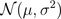, oùest inconnu.
Naturellement, on voudrait considérer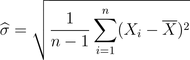On va poser, afin de simplifier un peu,et on va chercher à calculer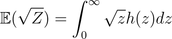où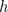est la densité de cette variable. Or on sait que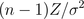suit une loi du chi-deux à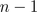degrés de liberté, dont la densité est la loi gamma suivante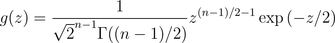En notant que l’on peut réécrire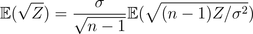on a que cette espérance s’écrit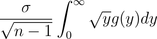soit en faisant un peu de calculs,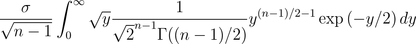ou encore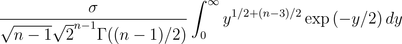Ce qui va se simplifier en utilisant la fonction gamma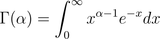On a ainsi